Also called Chebyshev Quadrature. A Gaussian Quadrature over the intervalwith Weighting Function. The Abscissas for quadrature orderare given by the roots of the Chebyshev Polynomial of the First Kind, which occur symmetrically about 0. The Weights are(1)

whereis the Coefficient ofin. For Hermite Polynomials,(2)

so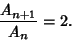(3)(4)

so(5)

Since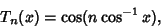(6)

the Abscissas are given explicitly by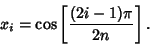(7)

Since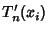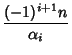(8)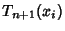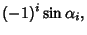(9)

where(10)

all the Weights are(11)

The explicit Formula is then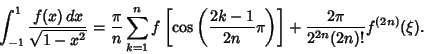(12)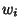2 ± 0.707107 1.5708 3 0 1.0472 ± 0.866025 1.0472 4 ± 0.382683 0.785398 ± 0.92388 0.785398 5 0 0.628319 ± 0.587785 0.628319 ± 0.951057 0.628319

References

Hildebrand, F. B. Introduction to Numerical Analysis. New York: McGraw-Hill, pp. 330-331, 1956.